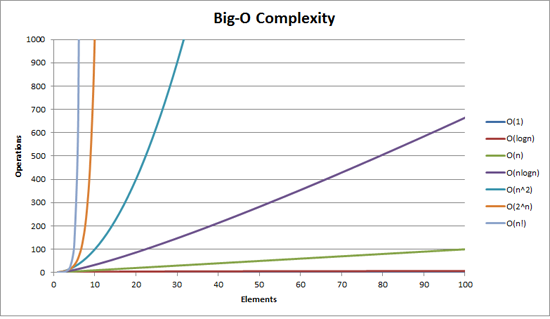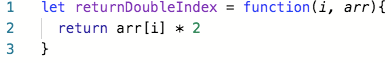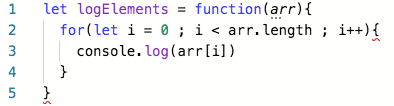# Big O Notation and Time/Space ComplexityBig O notation is a commonly used metric used in computer science to classify algorithms based on their time and space complexity.

# Big O Notation and Time/Space Complexity

Big O notation is a commonly used metric used in computer science to classify algorithms based on their time and space complexity. The time and space here is not based on the actual number of operations performed or amount of memory used per se, but rather how the algorithm would scale with an increase or decrease in the amount of data in the input. The notation will represent how an algorithm will run in the worst-case scenario- what is the maximum time or space an algorithm could use? The complexity is written as O(x) where x is the growth rate of the algorithm in regards to n, which is the amount of data input. Throughout the rest of this blog, input will be referred to as n.

# O(1)

O(1) is known as constant complexity. What this implies is that the amount of time or memory does not scale with n at all. For time complexity, this means that n is not iterated on or recursed- generally a value will be selected and returned or a value with be operated on and returned.Function that returns an index of an array doubled in O(1) time complexity.

For space, no data structures can be created that are a multiples of the size of n. Variables can be declared, but the number must not change with n.Function that logs every element in an array with O(1) space.

# O(logn)

O(logn) is known as logarithmic complexity. The logarithm in O(logn) has a base of 2. The best way to wrap your head around this is to remember the concept of halving: every time n increases by an amount k, the time or space increases by k/2. There are several common algorithms that are O(logn) a vast majority of the time to keep an eye out for: binary search, searching for a term in a binary search tree, and adding items to a heap.

## Big O Notation and Time Complexity

Big O notation is a simplified analysis of an algorithm’s efficiency. Big O notation gives us an algorithm’s complexity in terms.

## Big O Notation and Time Complexity

Big O notation is a simplified analysis of an algorithm’s efficiency. Big O notation gives us an algorithm’s complexity in terms of input size, N.

## Big O Notation: Calculating Time Complexity

Preparing for technical interviews can be difficult, frustrating, and confusing at times. As I continue my job search I thought I would take some time to share.

## The Big Confusion of Big O notation

The Big Confusion of Big O notation. Quick note, this is almost certainly confusing Big O notation (which is an upper bound) with Theta notation (which is a two-side bound).

## Time Complexity and Big O

Time Complexity and Big O. Understanding how algorithm efficiency is measured and optimized. In this article, we’re going to explore the concept of efficiency within computer science and learn some ways to measure and describe this efficiency.